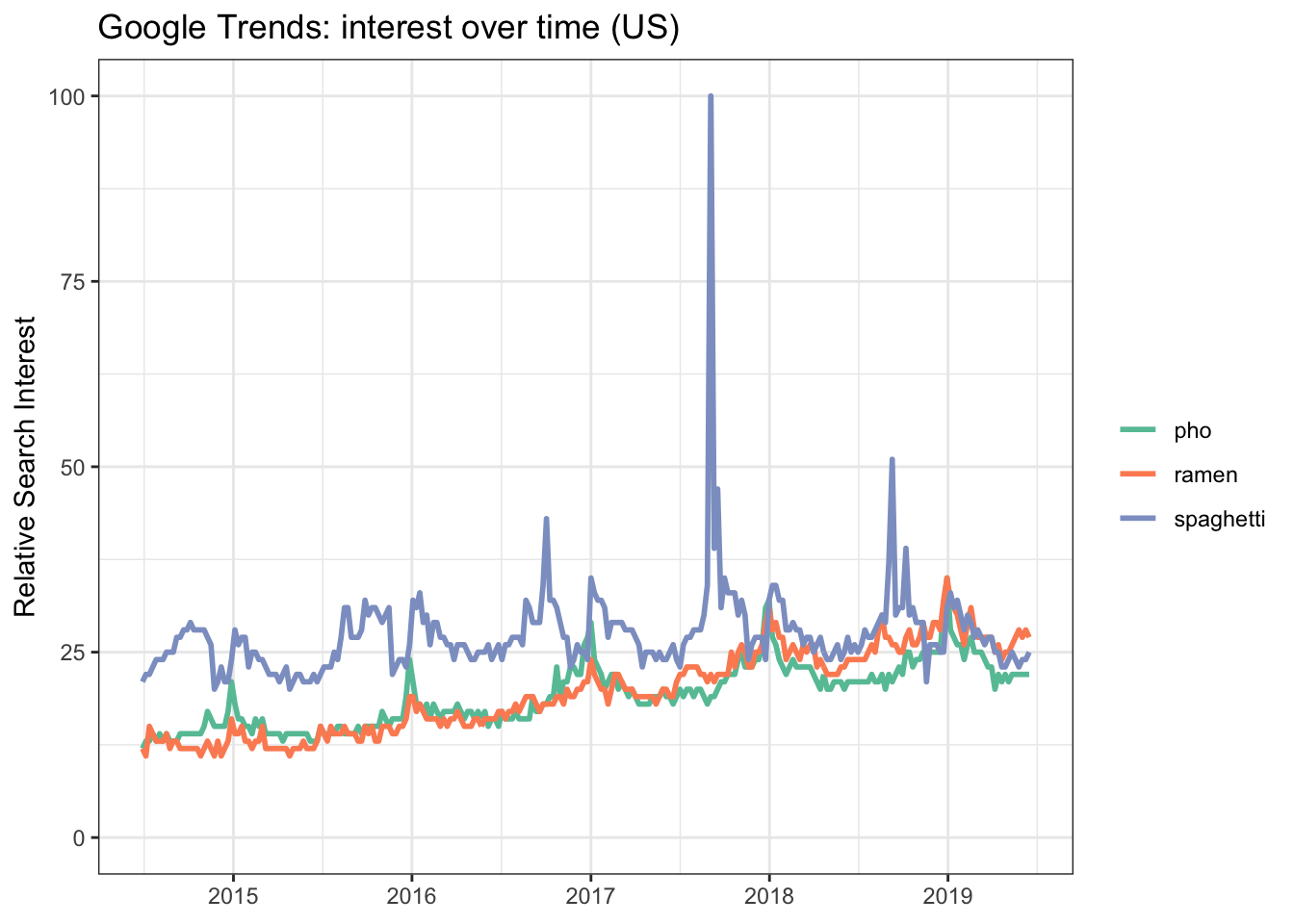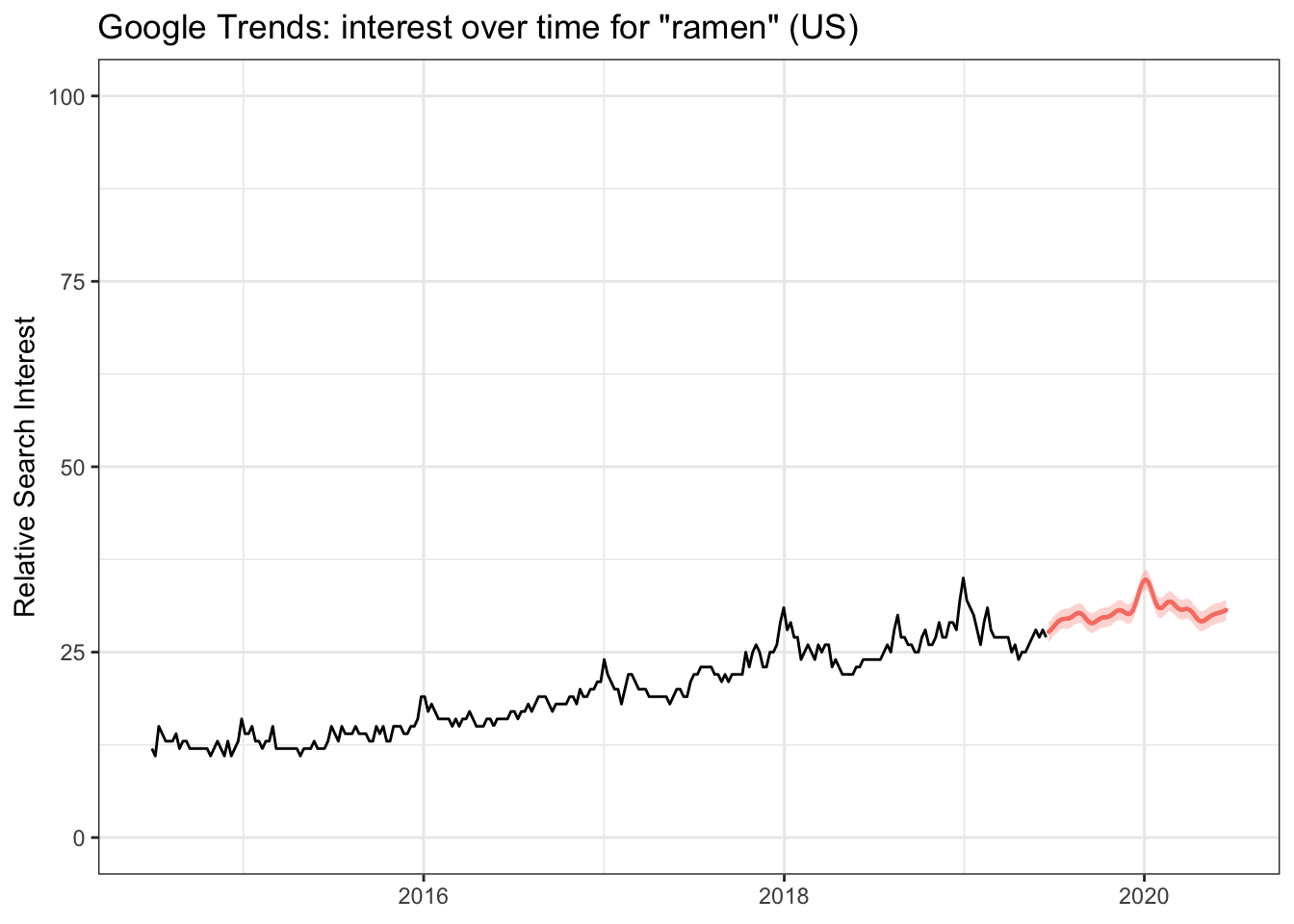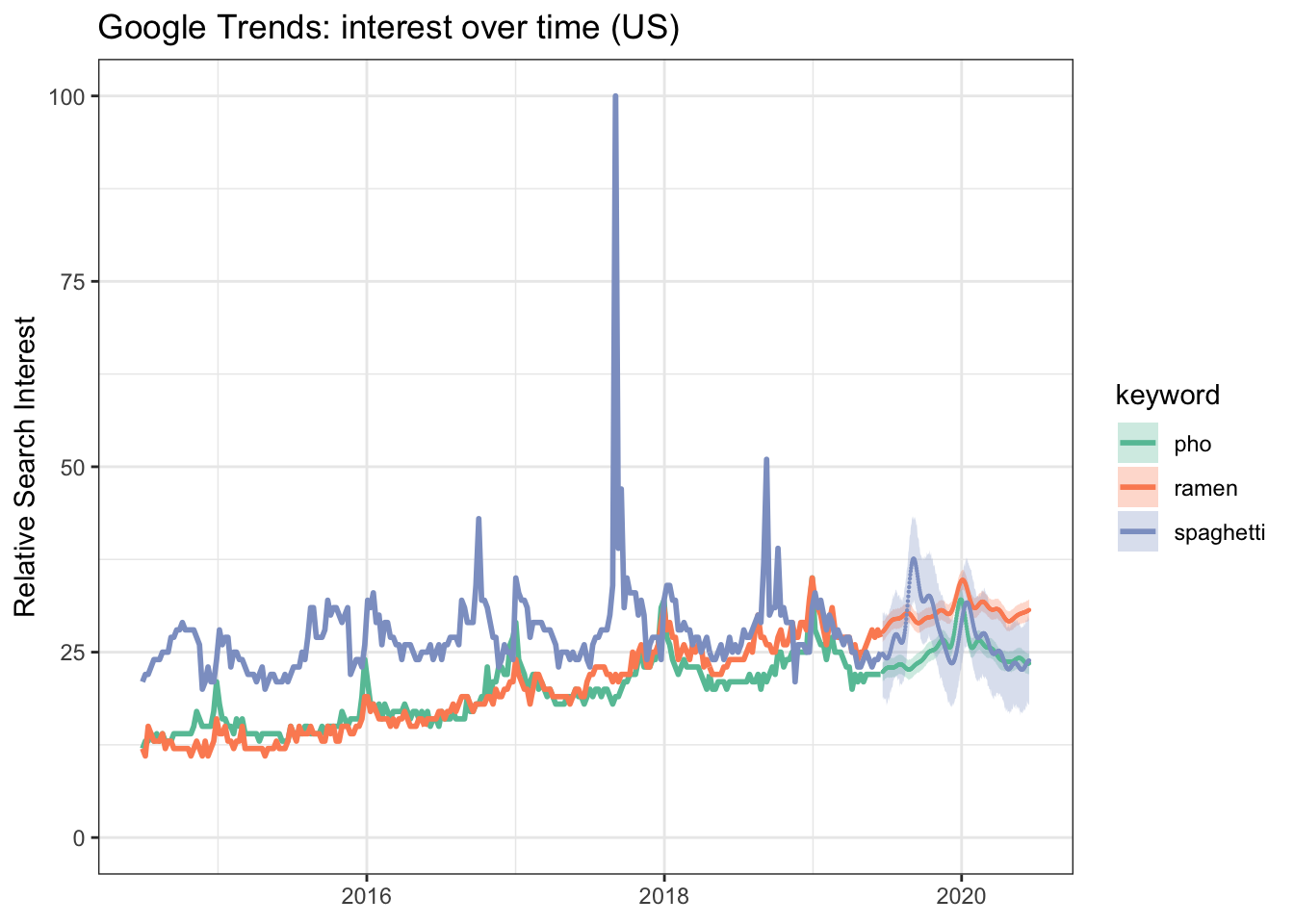Google Trends is great for understanding relative search popularity for a given keyword or phrase. However, if we wanted to explore the topics some more it is quite clunky to retrieve that data within the web interface.

Enter the gtrendsR package for #rstats and what better way to demonstrate how this works than by pulling search popularity for ramen, pho, and spaghetti (hot on the heels of my last article about ramen ratings)!

``````library(tidyverse)
library(lubridate)
library(gtrendsR)``````

## Clean up our dataframe

``````food_timeseries <- as_tibble(food\$interest_over_time) %>%
mutate(date = ymd(date)) %>% # CONVERT DATE FORMAT
filter(date < Sys.Date() - 7) # REMOVE "NOISY" DATA FROM LAST SEVEN DAYS``````

## Quick peek at data

``food_timeseries``
``````## # A tibble: 780 x 7
##    date        hits keyword geo   time      gprop category
##    <date>     <int> <chr>   <chr> <chr>     <chr>    <int>
##  1 2016-02-07    15 ramen   US    today+5-y web          0
##  2 2016-02-14    16 ramen   US    today+5-y web          0
##  3 2016-02-21    16 ramen   US    today+5-y web          0
##  4 2016-02-28    16 ramen   US    today+5-y web          0
##  5 2016-03-06    16 ramen   US    today+5-y web          0
##  6 2016-03-13    15 ramen   US    today+5-y web          0
##  7 2016-03-20    17 ramen   US    today+5-y web          0
##  8 2016-03-27    16 ramen   US    today+5-y web          0
##  9 2016-04-03    17 ramen   US    today+5-y web          0
## 10 2016-04-10    17 ramen   US    today+5-y web          0
## # … with 770 more rows``````

## Graph interest over time

``````food_timeseries %>%
ggplot() +
geom_line(aes(date, hits, color = keyword), size = 1) +
scale_y_continuous(limits = c(0, 100)) +
scale_color_brewer(palette = 'Set2') +
theme_bw() +
labs(x = NULL,
y = "Relative Search Interest",
color = NULL,
title = "Google Trends: interest over time (US)") ``````It looks like ramen has picked up traction over the last five years and even surpassed spaghetti popularity earlier this year.

I wonder what that will look like a year from now? We’ll look to using the prophet package from Facebook to forecast future popularity.

# Forecasting relative search popularity

``library(prophet)``

## Prepare the data

Let’s see how we do for ramen search popularity.

``````ramen_timeseries <- food_timeseries %>%
filter(keyword == 'ramen') %>%
select(date, hits) %>%
mutate(date = ymd(date)) %>%
rename(ds = date, y = hits) %>% # CONVERT COLUMN HEADERS FOR PROPHET
arrange(ds) # ARRANGE BY DATE``````

## Build the model

``````ramen_m <- prophet(ramen_timeseries)
ramen_future <- make_future_dataframe(ramen_m, periods = 365) # PREDICT 365 DAYS
ramen_ftdata <- as_tibble(predict(ramen_m, ramen_future))``````

## Combine forecast with actuals

``````ramen_forecast <- ramen_ftdata %>%
mutate(ds = ymd(ds),
segment = case_when(ds > Sys.Date()-7 ~ 'forecast',
TRUE ~ 'actual'), # SEGMENT ACTUAL VS FORECAST DATA
keyword = paste0("ramen")) %>%
select(ds, segment, yhat_lower, yhat, yhat_upper, keyword) %>%
left_join(ramen_timeseries) # JOIN ACTUAL DATA``````

## Plot forecasting results

``````ramen_forecast %>%
rename(date = ds,
actual = y) %>%
ggplot() +
geom_line(aes(date, actual)) + # PLOT ACTUALS DATA
geom_point(data = subset(ramen_forecast, segment == 'forecast'),
aes(ds, yhat), color = 'salmon', size = 0.1) + # PLOT PREDICTION DATA
geom_ribbon(data = subset(ramen_forecast, segment == 'forecast'),
aes(ds, ymin = yhat_lower, ymax = yhat_upper),
fill = 'salmon', alpha = 0.3) + # SHADE PREDICTION DATA REGION
scale_y_continuous(limits = c(0,100)) +
theme_bw() +
labs(x = NULL, y = "Relative Search Interest",
title = "Google Trends: interest over time for \"ramen\" (US)")``````The chart above doesn’t look too bad however this is relative search popularity so we need to compare the prediction with pho and spaghetti as well.

``````# FUTURE NOTE: REFACTOR FOR DRY PRINCIPLES

# BUILD FORECASTING MODEL FOR PHO
pho_timeseries <- food_timeseries %>%
filter(keyword == 'pho') %>%
select(date, hits) %>%
mutate(date = ymd(date)) %>%
rename(ds = date, y = hits) %>%
arrange(ds)

pho_m <- prophet(pho_timeseries)
pho_future <- make_future_dataframe(pho_m, periods = 365)
pho_ftdata <- as_tibble(predict(pho_m, pho_future))

pho_forecast <- pho_ftdata %>%
mutate(ds = ymd(ds),
segment = case_when(ds > Sys.Date()-7 ~ 'forecast',
TRUE ~ 'actual'),
keyword = paste0("pho")) %>%
select(ds, segment, yhat_lower, yhat, yhat_upper, keyword) %>%
left_join(pho_timeseries)

# BUILD FORECASTING MODEL FOR SPAGHETTI
spaghetti_timeseries <- food_timeseries %>%
filter(keyword == 'spaghetti') %>%
select(date, hits) %>%
mutate(date = ymd(date)) %>%
rename(ds = date, y = hits) %>%
arrange(ds)

spaghetti_m <- prophet(spaghetti_timeseries)
spaghetti_future <- make_future_dataframe(spaghetti_m, periods = 365)
spaghetti_ftdata <- as_tibble(predict(spaghetti_m, spaghetti_future))

spaghetti_forecast <- spaghetti_ftdata %>%
mutate(ds = ymd(ds),
segment = case_when(ds > Sys.Date()-7 ~ 'forecast',
TRUE ~ 'actual'),
keyword = paste0("spaghetti")) %>%
select(ds, segment, yhat_lower, yhat, yhat_upper, keyword) %>%
left_join(spaghetti_timeseries)

# COMBINE ALL MODELS
keyword_forecast <- rbind(ramen_forecast, pho_forecast, spaghetti_forecast) %>%
rename(date = ds, actual = y)``````

## Final plot

``````keyword_forecast %>%
ggplot() +
geom_line(aes(date, actual, color = keyword), size = 1) +
geom_ribbon(data = subset(keyword_forecast, segment == 'forecast'),
aes(date, ymin = yhat_lower, ymax = yhat_upper, fill = keyword),
alpha = 0.3) +
geom_point(data = subset(keyword_forecast, segment == 'forecast'),
aes(date, yhat, color = keyword), size = 0.1) +
scale_y_continuous(limits = c(0,100)) +
scale_color_brewer(palette = 'Set2') +
scale_fill_brewer(palette = 'Set2') +
theme_bw() +
labs(x = NULL, y = "Relative Search Interest",
title = "Google Trends: interest over time (US)") ``````One year from now we should expect to see ramen at the top followed by pho and spaghetti fighting for a close second in terms of relative search interest.